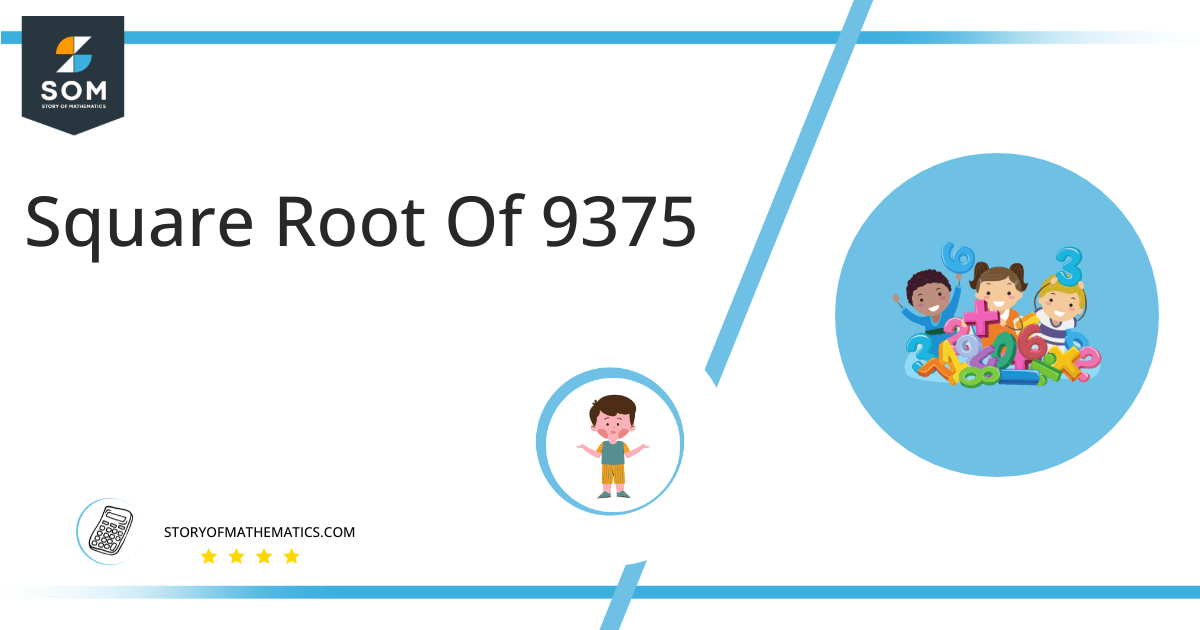# Square Root of 9375 + Solution With Free StepsThe symbol indicates the square root, and 9375’s square root is equivalent to 96.82 when written as √9375. Therefore, the power of 1/2 is equal to the square root. The following sentences explain various approaches to finding the square root of 9375 are illustrated.

In this article, we will analyze and find the square root of 9375 using various mathematical techniques, such as the approximation method and the long division method.

## What Is the Square Root Of 9375?

The square root of the number 9375 is 96.82.

The square root can be defined as the quantity that can be doubled to produce the square of that similar quantity. In simple words, it can be explained as:

√9375 = √(y96.82 x 96.82)

√9375 = √(96.82)$^2$

√9375 = ±96.82

The square can be canceled with the square root as it is equivalent to 1/2; therefore, obtaining 96.82. Hence 96.82 is 9375’s square root. The square root generates both positive and negative integers.

## How To Calculate the Square Root of 9375?

You can calculate the square root of 9375 using any of two vastly used techniques in mathematics; one is the Approximation technique, and the other is the Long Division method.

The symbol √ is interpreted as 9375 raised to the power 1/2. So any number, when multiplied by itself, produces its square, and when the square root of any squared number is taken, it produces the actual number.

Let us discuss each of them to understand the concepts better.

### Square Root of 9375 by Long Division Method

The process of long division is one of the most common methods used to find the square roots of a given number. It is easy to comprehend and provides more reliable and accurate answers. The long division method reduces a multi-digit number to its equal parts.

Learning how to find the square root of a number is easy with the long division method. All you need are five primary operations- divide, multiply, subtract, bring down or raise, then repeat.

Following are the simple steps that must be followed to find the square root of 9375 using the long division method:

### Step 1

First, write the given number 9375 in the division symbol, as shown in figure 1.

### Step 2

Starting from the right side of the number, divide the number 9375 into pairs such as 75 and 93.

### Step 3

Now divide the digit 93 by a number, giving a number either 9 or less than 9. Therefore, in this case, the remainder is 12 whereas the quotient is 9.

### Step 4

After this, bring down the next pair 75. Now the dividend is 1275. To find the next divisor, we need to double our quotient obtained before. Doubling 9 gives 18; hence consider it as the next divisor.

### Step 5

Now pair 2 with another number to make a new divisor that results in $\leq$ 1275 when multiplied with the divisor. If the number is not a perfect square, add pair of zeros to the right of the number before starting division.

### Step 6

Adding 6 to the divisor and multiplying 186 with 6 results in 1116 < 1275. The remainder obtained is 159. Move the next pair of zeros down and repeat the same process mentioned above.

### Step 7

Keep on repeating the same steps till the zero remainder is obtained or if the division process continues infinitely, solve to two decimal places.

### Step 8

The resulting quotient 96.82 is the square root of 9375. Figure 1 given below shows the long division process in detail:Figure 1

### Square Root by Approximation Method

The approximation method involves guessing the square root of the non-perfect square number by dividing it by the perfect square lesser or greater than that number and taking the average.

The given detailed steps must be followed to find the square root of 9375 using the approximation technique.

### Step 1

Consider a perfect square number 96 less than 9375.

### Step 2

Now divide 9375 by 96.

9375 ÷ 96 = 97.65

### Step 3

Now take the average of 96 and 97.65. The resulting number is approximately equivalent to the square root of 9375.

(96 + 97.65) ÷ 2 = 96.82

### Important points

• The number 9375 is not a perfect square.
• The number 9375 is an irrational number.
• The number 9375 can be split into its prime factorization.

## Is Square Root of 9375 a Perfect Square?

The number 9375 is not a perfect square. A number is a perfect square if it splits into two equal parts or identical whole numbers. If a number is a perfect square, it is also rational.

A number expressed in p/q form is called a rational number. All the natural numbers are rational. A square root of a perfect square is a whole number; therefore, a perfect square is a rational number.

A number that is not a perfect square is irrational as it is a decimal number. As far as 9375 is concerned, it is not a perfect square. It can be proved as below

Factorization of 9375 results in 5 x 1875.

Taking the square root of the above expression gives:

= √(5 x 1875)

= (5 x 1875)$^{1/2}$

= 96.82

This shows that 9375 is not a perfect square as it has decimal places; hence it is an irrational number.

Therefore the above discussion proves that the square root of 9375 is equivalent to 96.82.Images/mathematical drawings are created with GeoGebra.